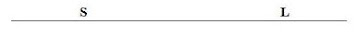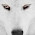`“Life is like riding a bicycle.  To keep your balance you must keep moving.”–Albert Einstein`

Saturday, August 16, 2014

AP Physics 1 and 2 – Multiple Choice Practice Questions

“One of the deep secrets of life is that all that is really worth the doing is what we do for others.”
– Lewis Carroll

As you know, with effect from the academic year 2014 – 2015, AP Physics B course is discontinued and is replaced by two new courses, namely AP Physics 1 and AP Physics 2. Both courses are algebra based. It is hoped that the new courses will help the students to explore deeper into key concepts in physics both in theory and practice.

Today we shall discuss a few multiple choice practice questions relevant to AP Physics 1 and AP Physics 2 courses. Questions (1), (2) and (3) are for AP Physics 1 where as questions (4) , (5) and (6) are for AP Physics 2.

Questions (1) and (2) are single correct answer type multiple choice questions where as question (3) is multi-correct answer type multiple choice question.

Similarly questions (4) and (5) are single correct answer type multiple choice questions where as question (6) is multi-correct answer type multiple choice question.

(1) The magnitude of the gravitational force on a solid sphere A of radius R and density d at a point P in the earth’s atmosphere is F. If this sphere is replaced by another solid sphere B of radius 2R and density 2d, the magnitude of the gravitational force acting on it will be

(a) 2F

(b) 4F

(c) 8F

(d) 16F

(2) The sphere A in question number (1) starts to fall freely under earth’s gravity with an acceleration of magnitude g1. What will be the magnitude of the acceleration of free fall of sphere B under earth’s gravity?

(a) g1

(b) 2g1

(c) 4g1

(d) 16g1

(3) A loud speaker is excited with an alternating current of frequency 1000 Hz. The sound produced by the loud speaker propagates in air along the x-axis through points P and Q (Fig.), which lie 5 m apart. Which of the following statements are true in this context?

(a) Air molecules at P and Q vibrate parallel to the x-axis

(b) The speed of sound at Q is less than the speed at P since Q is at a greater distance from the loud speaker.

(c) Air molecules at P are carried along the x-axis to the point Q and then farther away from Q.

(d) Air molecules at P and Q always maintain their mean positions at P and Q.

(4) An electron is projected with uniform velocity v along the axis of a plane circular coil carrying a steady current. Which one among the following statements is correct?
(a) The electron will continue to move along the axis with uniform acceleration
(b) The electron will move along a helical path around the axis of the circular coil.
(c) The electron will continue to move along the axis with uniform acceleration or deceleration, depending on the direction of the current in the coil
(d) The electron will continue to move along the axis with uniform velocity v

(5) A beam of sun light is allowed to fall normally on one face of a glass slab of uniform thickness. Light of which component colour will emerge last through the opposite face of the slab?
(a) Green
(b) Red
(c) Violet
(d) All colours will emerge simultaneously(6) S is a police vehicle which sounds a siren and L is a listener in a car on a straight road. In which of the following cases will the listener L note an apparent increase in the frequency of the sound emitted by the siren?

(a) S and L moving in the direction SL with speeds 2u and u respectively.

(b) S and L moving in the direction LS with speeds u and 2u respectively.

(c) S and L moving in the direction SL with speeds u and 2u respectively

(d) S and L stationary and wind blowing in the direction SL with speed u.

Now, let us answer the above questions:
(1) The gravitational force is directly proportional to the mass of the sphere. The mass of sphere A is (4/3) πR3d where as the mass of sphere B is (4/3) π(2R)3(2d) = 16(4/3) πR3d.
Since the mass of sphere B is 16 times the mass of A the gravitational force on B is 16 times that on A. The answer is 16F [Option (d)].

(2) Acceleration = Force/Mass.
Even though the gravitational force on sphere B is 16 times that on sphere A, the acceleration of sphere B is the same as that of sphere A since the mass of sphere B is 16 times that of sphere A. The answer is g1 [Option (a)].

(3) Sound is a longitudinal wave. In longitudinal waves particles of the medium vibrate parallel to the direction of propagation of the wave. Option (a) is correct. When a wave propagates through a medium, particles of the medium just vibrate about their position and are not carried along with the wave. Therefore, option (d) is correct and (c) is incorrect. The speed of a wave depends only on the properties of the medium through which the wave propagates. Therefore, option (b) is incorrect.

(4) The magnetic field due to a current carrying circular coil at any point on its axis is directed along the axis. Therefore, the electron projected along the axis of the coil moves either parallel or anti-parallel to the direction of the magnetic field produced by the coil. Consequently the magnetic force on the electron is zero.
[The expression for the magnitude of the magnetic force F on a moving charge is F = qvB sinθ where q is the charge, v is the speed of the charge, B is the magnitude of the magnetic flux density and θ is the angle between the direction of motion of the charge and the direction of the magnetic field. In the present case θ is either zero or 180º so that sinθ is zero].
The electron will therefore be unaffected and will continue to move with uniform velocity v [Option (d)].

(5) Violet light has the least speed in a material medium. Therefore, light of violet colour will emerge last from the opposite face of the slab [Option (c)].
[Note that the refractive index of a material medium for violet light is maximum (since violet light is bent most). Since the refractive index of a medium is the ratio of the speed of light in free space to the speed in the medium, it follows that the speed is smaller if the refractive index is greater].

(6) This question involves Doppler effect, which is the phenomenon by which the frequency of a wave as measured by an observer is changed because of the motion of the source, observer and the medium.
Let the source S of sound (fig.) move with velocity vS, the listener move with velocity vL and the wind blow with velocity w, all in the same direction as shown in the figure.

Wind→ w         S●→vS        L●→vL

The apparent frequency (n’) of sound is then given by
n’ = n(v + w vL)/ (v + w vS) ………………………(i)
where n is the real frequency of the sound and v is the velocity of sound. It may be noted that the above relation has been derived on the assumption that the source is moving towards the listener, the listener is moving away from the source and the wind is blowing from the source to the listener.
Considering case (a), we have n’ = n(v + 0 u)/ (v + 0 2u) so that n’ > n
Considering case (b), we have n’ = n(v + 0 + 2u)/ (v + 0 + u) so that n’ > n
[Note that the signs of vL and vS are negative in this case]
Considering case (c), we have n’ = n(v + 0 – 2u)/ (v + 0 u) so that n’ < n
Considering case (d), we have n’ = n(v + w – 0)/ (v + w – 0) so that n’ = n
Thus cases (a) and (b) are the correct options.
[Even if you don’t remember the above expression (i), you will be able to answer many questions if you note that the apparent frequency increases if the source moves towards the listener (relatively) or the listener moves towards the source (relatively). If they move away from each other, the apparent frequency decreases].

You can access all posts on waves on this site by clicking on the label ‘wave motion (including sound)’

1 comment:

1.As nice blog,but you could have made this blog more cool if you could configure the language changer pluigin but all the same you havehttp://www.iessaywriter.com done a good job:))